# PSEB 8th Class Science Solutions Chapter 11 Force and Pressure

## PSEB Solutions for Class 8 Science Chapter 11 Force and Pressure

PSEB 8th Class Science Guide Force and Pressure Textbook Questions and Answers

Exercises

Question 1.
Give two examples each of situations in which you push or pull to change the state of motion of objects.

1. In a cricket game, fielders stop the ball hit by a batsman.
2. Brakes applied to stop a moving vehicle.

Question 2.
Give two examples of situations in which applied force causes a change in the shape of an object.

1. Pressing a rubber ball placed on a table.
2. Spring fixed in the seat of bicycle pressed down due to weight of the rider.

Question 3.
Fill in the blanks in the following statements:
[а] To draw water from a well we have to ………………. at the rope.
[b] A charged body …………….. an uncharged body towards it.
[c] To move a loaded trolley we have to …………………. it.
[d] The north pole of a magnet …………… the north pole of another magnet.
(a) pull
(b) attracts
(c) push
(d) repels.Question 4.
An archer stretches her bow while taking aim at the target. She then releases the arrow, which begins to move towards the target. Based on this information fill up the gaps in the following statements using the following terms.
Muscular, contact, non-contact, gravity, friction, shape, attraction.
[а] To stretch the bow, the archer applies a force that causes change in its …………………. .
[b] The force applied by the archer to stretch the bow is an example of ………………… force.
[c] The type of force responsible for a change in the state of motion of the arrow is an example of a ………………. force.
[d] While the arrow moves towards the target, the forces acting on it are due to ………………….. and that due to ………………….. of air.
(a) shape,
(b) muscular,
(c) muscular,
(d) gravity, friction.

Question 5.
In the following situations identify the agent exerting the force and the object on which it acts. State the effect of force in each case.
[a] Squeezing a piece of lemon between the fingers to extract its juice.
[b] Taking out paste from a toothpaste tube.
[c] A load suspended from a spring while its other end is on a hook fixed to a wall.
[d] An athlete making a high jump to clear the bar at a certain height.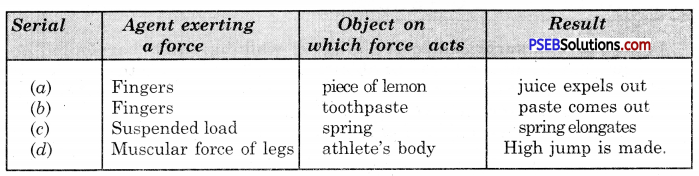Question 6.
A blacksmith hammers a hot piece of iron while making a tool. How does the force due to hammering affect the piece of iron ?
The force applied by the hammer flattens the piece of iron i.e. changes its shape and size.

Question 7.
An inflated balloon was pressed against a wall after it has been rubbed with a piece of synthetic cloth. It was found that the balloon sticks to the wall. What force might be responsible for attraction between the balloon and the wall.
Electrostatic force.

Question 8.
Name the forces acting on a plastic bucket containing water held above ground level in your hand. Discuss why the forces acting on the bucket do not bring a change in its state of motion.
Forces acting on plastic bucket

1. Force of gravity acting downwards.
2. Muscular force of arms acting upwards.

The two forces do not bring about any change in state of motion of bucket because both forces are equal and act in the opposite direction and thus cancel each other’s effect.

Question 9.
A rocket has been fired upwards to launch a satellite in its orbit. Name the two forces acting on the rocket immediately after leaving the launching pad.
When a rocket leaves launching pad, the following forces act on it:

1. Gravitational force of earth (downwards).
2. Propelling force of gases emitted by combustion of fuels (upwards).Question 10.
When we press the bulb of a dropper with its nozzle kept in water, air in the dropper is seen to escape in the form of bubbles. Once we release the pressure on the bulb, water gets filled in the dropper. The rise of water in the dropper is due to
(a) pressure of Water
(b) gravity of the earth
(c) shape of rubber bulb
(d) atmospheric pressure.
(d) atmospheric pressure.

PSEB Solutions for Class 8 Science Force and Pressure Important Questions and Answers

Multiple Choice Questions

Question 1.
Raman was surprised to see a camel walking easily barefooted on sand but he himself was unable to walk barefoot on sand. What is the reason behind this ?
(a) The surface area of the foot of the camel is more
(b) The surface area of the foot of Raman is more.
(c) Both (a) and (b)
(d) None of the above.
(a) The surface area of the foot of the camel is more.

Question 2.
By exerting force on anything:
(a) results in increasing the speed of the object
(b) results in decreasing the speed of the object
(c) Results in change in the direction of the motion of the object
(d) All the effects are possible.
(d) All the effects are possible.

Question 3.
Force is:
(a) a push on an object
(b) a pull on an object
(c) a push or pull on an object
(d) neither a push nor a pull.
(c) a push or pull on an object.

Question 4.
The food in the foodpipe is pushed forward during digestive process :
(a) By friction force
(b) Muscular force
(c) Contact force
(d) All of these.
(b) Muscular force.

Question 5.
The force of friction on a moving object always acts:
(a) in the direction of motion
(b) opposite to the direction of motion
(c) in the direction of motion upwards
(d) opposite to the direction of motion downwards
(b) opposite to the direction of motion.Question 6.
While carrying luggage on the railway station a ‘coolie’ often rolls his cloth and places it on his head to :
(a) increase force
(b) increase pressure
(c) reduce weight
(d) reduce pressure
(d) reduce pressure.

Question 7.
The pressure applied by liquids …………………. with the increase in depth.
(a) decreases
(b) remains same
(c) increases
(d) depends on the nature of liquids
(c) increases.

Question 1.
What is common in following actions :
kicking, hitting, lifting, pulling, etc ?
In all above actions, force is used to change the motion of the object.

Question 2.
What other terms are used for actions involving motion of objects ?
Push or pull.

Question 3.
What is force ?
Force. A push or pull that changes or tends to change the state, shape or direction of motion of the body is called force.

Question 4.
What is meant by pressure ?
Pressure. Force acting per unit area is called a pressure.

Question 5.
Explain with an example that force can change the speed of an object.
Suppose you are going on your bicycle with a speed of 10 m/s in north to south direction, and if your friend gives a strong push to your bicycle in the same direction then the speed of your bicycle will definitely increase.Question 6.
Give an illustration to show that a force can change the shape of the object.
If a rubber ball is pressed in between the two palms, we find that ball is no longer round but becomes oblong.

Question 7.
Give an illustration to show that a force can produce change in both the speed and direction of motion.
When the batsman plays the ball thrown by bowler, the speed and direction of the ball changes.

Question 8.
Give an illustration to show that force can change the direction of mofion of an object.
In game of football, we can change the direction of a moving football by kicking it.

Question 9.
What causes force to act ?
Interaction between two objects.

Question 10.
What happens when force is applied in the direction of motion ?
The speed of object increases.

Question 11.
What happens when forces acting on an object are in opposite direction and equal ?
Net force will be zero i.e. object will not move in any direction.Question 12.
What is contact force ?
Contact force. The force which acts only, when objects are in contact, is called contact force.

Question 13.
Give examples of contact force.
Muscular and frictional forces.

Question 14.
Which type of force is force of gravity ?
Non-contact force.

Question 15.
Give an example of non-contact force.
Magnetic force.

Question 16.
What is electrostatic force ?
Electrostatic force. The force exerted by a charged body is called electrostatic force.

Question 17.
How can pressure be increased or decreased ?
By changing the area on which force acts.Question 18.
Why is the foundation of wall made wider ?
To decrease the pressure of the walls on the base.

Question 19.
What is relation between force, area and pressure ?
Pressure = Force/Area.

Question 20.
Which type of tool is needed for cutting or piercing ?
A sharp edged tool.

Question 1.
What is done to stop a moving ball ?
To stop a moving ball, equal force is applied in the direction opposite to the direction of motion of the ball.

Question 2.
Can a moving object on a smooth surface stop by itself ? If so, why ?
A moving object can stop by itself due to friction between it and the surface on which it is moving. Friction acts in the opposite direction thus, stops the ball.

Question 3.
When is net force applied zero ? Give an example.
When two forces are applied in opposite direction and are equal in magnitude then net force is zero. Example : A game of tug-of-war.

Question 4.
Force is a vector quantity. How ?
A vector quantity is that quantity which can be represented completely by magnitude as well as direction. To represent the force magnitude as well as direction both are required. Hence force is a vector quantity.Question 5.
Name various types of forces.
The following are the various types of forces:

1. Muscular force.
2. Magnetic force.
3. Electrostatic force.
4. Gravitational force.
5. Frictional force.

Question 6.
List two effects of force.
Effects of Force.

1. Force changes the state of motion.
2. Force changes the shape of an object.

Question 7.
What is state of motion of an object ?
State of motion of an object is its speed and direction of motion. The state of rest is when speed is zero. The change in speed or direction of motion or both means a change in state of motion of an object.

Question 8.
Can force change only the direction of motion without any change in speed of an object ? If yes, how ?
Yes, force can change only direction of motion without any change in speed of an object. It can be shown as follows:
Experiment.
Take a small stone. Tie it to a string. Whirl string in circular path with your hand. It is noted that stone moves with a constant speed in circular path. But when the whirling is stopped, the stone tries to move in horizontal path. This shows that force applied is used to change the direction of motion of the stone.

Question 9.
Give few examples of muscular force.
Muscular force. Walking, breathing, running, lifting, sking, fighting are few activities which require muscular force to accomplish.

Question 10.
Give an example of contact force.
When a moving striker collides with a disc on a carrom board, the disc begins to move showing the effect of contact force.Question 11.
Give two examples of non-contact forces.
Examples of non-contact force.

1. When two magnets lie side by side at a distance they either repel or attract each other.
2. A charged body can attract or repel other uncharged or charged body even from a distance.

Question 12.
Why is force of gravity termed as non-contact force ? Explain.
Force of gravity acts on objects lying on or near the surface of earth. It also acts on distant objects such as shown by leaves falling from trees, water in pipes flowing downwards, revolution of moon around the earth. In all cases, objects are not in contact with the earth. So it is termed as a non-contact force.

Question 13.
Define pressure and what are its units ?
Pressure. The force acting per unit area of a surface, is called pressure. Force
Pressure = $$\frac{\text { Force }}{\text { Area }}$$
Unit of Pressure is Pascal or N m-2.

Question 14.
Why do you use sharp edged knife to cut a fruit ?
In the sharp edged knife the effect of force increases the pressure on a fruit and thus cut it easily.

Question 15.
Where is pressure greater and the least inside a bottle filled with water ?
In a bottle filled with water, the pressure is the greatest at the bottom of the bottle and it is the least at the top of the bottle.

Question 16.
What makes a balloon stretch as it is filled with air ?
When we fill air in a balloon, the air exerts pressure on inner surface of the balloon. The pressure of the air inside the balloon stretches it.Question 17.
Show experimentally that pressure increases with the depth.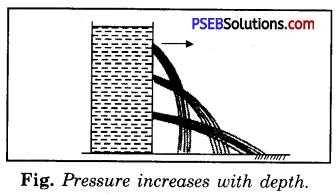The pressure of a liquid depends upon its depth. This can be proved by an experiment given below :
Experiment.
Take a deep vessel having atleast three holes at different heights on one side of the vessel as shown in Fig. Fill it with water. We shall find that the jet of water from the lowest hole reaches the farthest. This proves that at the lowest hole, the force or pressure is
maximum. Fig. Pressure increases with depth.

Question 18.
What is atmospheric pressure ?
Atmospheric pressure. Our earth is surrounded by an air column. This air column like liquid columns exerts pressure on all the objects lying on earth. This pressure exerted by air of the atmosphere is called atmospheric pressure.

Question 19.
Atmospheric pressure is so great, why are we not crushed by it ?
Our body and the bodies of other organisms are made up of cells which have fluids that exert pressure from within. This pressure exerted from the inside of the cell is equal to the atmospheric pressure and prevents us from being crushed.

Question 20.
The following picture shows the sheet of rubber tied to one side of the pipe filled with water. If the level of water is raised then what will be the effect on balloon of rubber. Give reason.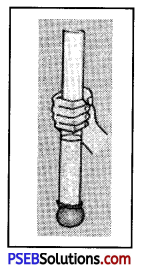When the level of water is raised in pipe, the rubber sheet tied to the pipe blows. This happens because with the raise of level of water, the pressure exerted on rubber increases.
That is way, rubber sheet blows.

Question 1.
What are the different types of forces ? Give an example of each.
Types of forces:
The following are the different types of forces:
1. Muscular force
2. Magnetic force
3. Electrostatic force
4. Gravitational force
5. Frictional force.

1. Muscular force:
The force applied by a living being with its muscles is known as muscular force e.g., bullocks apply muscular force to draw a cart.

2. Magnetic force:
A magnet has the property of attracting things made of cobalt, nickel, iron or steel. The force produced by a magnetic substance is called the magnetic force e.g., a magnet can move small iron pins.

3. Electrostatic force:
When substances like plastic and terylene are rubbed against each other, charge is excited on them. The force produced due to this electricity is called the electrostatic force e.g., when a glass rod is rubbed with silk, the rod moves bits of paper due to electrostatic force.

4. Gravitational force:
The earth exerts a force of attraction on all things on its surface and beyond. The force of attraction exerted by the earth is called the gravitational force or force due to gravity. An object dropped from a certain height falls on the earth due to the gravitational force.

5. Frictional force:
When an object moves along some other object, the force which acts between the surfaces of a contact of the two objects is called the frictional force. The frictional force opposes the motion of the object e.g., a marble rolled on the ground stops after sometime due to the frictional force.Question 2.
When a force is applied to a body, state its effect.
The following conclusion can be drawn about the effect of force applied to an object:

1. Change in speed : The applied force can produce a change in the speed of an object.
2. Change in direction of motion : The applied force can change the direction of motion of object.
3. Change in both speed and direction of motion : The applied force can produce a change in both the speed and direction of motion.
4. Change in shape and size of the body : The applied force can change the shape and size of an object.

Question 3.

1. Force can move a stationary object. For example, a toy can be moved by applying force.
2. It can slow down a moving object. For example, a bicycle slows down on applying force due to brakes.
3. It can change the direction of motion of an object. For example* a batsman hits the ball and changes its direction.
4. It can change the shape of an object. For example, squeezing a sponge changes its shape.

1. Frictional force is responsible for wear and tear of tyres of vehicles and of our shoes.
2. Frictional force also generates heat. This can be harmful. The heat produced in moving machines reduces their efficiency.

Question 4.
Describe an experiment to show that pressure is the same at all points at the same depth.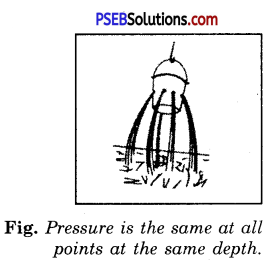Experiment.
Take an empty tin can and pierce several holes of equal size at the same height and cover them with cellotape to be opened at will. Now fill the can up to its brim and remove the cellotape in order to open the holes (Fig.). It will be found that water from all the holes traverses equal horizontal distance. This is because of the fact that the pressure at all points is the same at the same depth.

Question 5.
How can you demonstrate the presence of atmospheric pressure experimentally ?
Experiment.
Take a metallic can. Add little water into it. Remove its cap and heat the can, as shown in Fig. (a). The water boils and steam starts coming out from the mouth of the can. The steam forces out most of the air from the can. Now close the can with an air tight lid. Pour cold water on the can. Due to the cold water the steam inside the can condenses to liquid state. A partial vacuum is created. The external pressure (atmospheric) becomes greater than the inside pressure of steam. Hence high external pressure crushes the container [See Fig. (b)].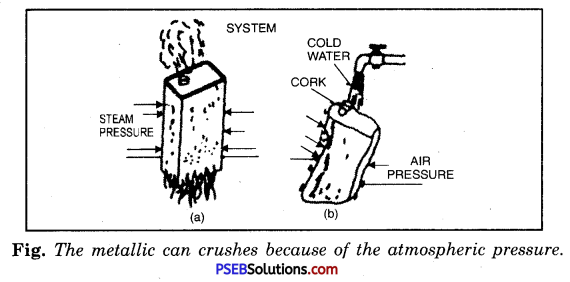Force and Pressure PSEB 8th Class Science Notes

• Force is a push or a pull which produces or tends to produce change in the state of a body.
• Effect of force depends not only on the magnitude of force but also depends on the area on which it is applied.
• Force can change the speed, the direction of motion and the shape of an object,
• There are various types of forces such as muscular, magnetic, electrostatic, gravitational and frictional.
• Force acting per unit area is called pressure.
• Liquids and gases apply pressure on the surfaces of the container.
• Atmosphere also exerts pressure, called atmospheric pressure. ‘
• The pressure exerted by liquids at a given depth is equal in all directions.
• Pressure of column of liquid depends on its depth. The more the depth of liquid column, the more is the pressure.
• Gases exert pressure in all directions.
• Force: It is defined as an external cause which changes or tends to change the state of rest or of uniform motion of an object. It is a vector quantity.
• Resultant Force: When two or more forces act on a body simultaneously, then a single force which produces the same effect as the combined effect of all the forces together, is called resultant force.
• Force of Friction: The force which opposes the motion of one body over another body in contact with it, is called the force of friction or simply friction.
• Gravitation: Gravitation is the attraction between any two bodies (particles) in the universe.
• Weight: Weight of a body is the force with which the body is attracted towards the centre of the earth.
• Pressure: Force acting per unit area is called pressure.
• Atmospheric Pressure: Pressure exerted by atmosphere on all the objects lying on the earth is called atmospheric pressure.

Punjab State Board PSEB 8th Class Science Book Solutions Chapter 11 Force and Pressure Textbook Exercise Questions and Answers.